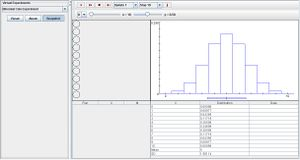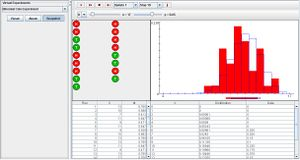# Difference between revisions of "SOCR EduMaterials Activities BinomialCoinExperiment"

## Description

The binomial coin experiment performs n coin tosses with the probability of heads p for each toss. In the data table below, the number of heads X and the proportion of heads M are updated after every trial. The experimenter has the ability to select either X or M, and modifying parameters n and p with the scroll bars above. The graph illustrates the discrete probability density function and moments of the selected variable as blue and the empirical probability density function and moments of the selected variable are shown in red. To the right of the data table is the numerical values of the distribution table that also updates the mean and standard deviation of the gathered data in the experiment.

## Goal

To provide a simulation for natural occurring phenomena with two distinct qualitative variables (e.g. heads/tails, up/down, true/false, etc.) and develop a general foundation about binomial events.

*Experiment:

Go to the SOCR Experiments [] and select the Binomial Coin Experiment from the drop-down list of experiments on the top left. The image below shows the initial view of this experiment:When pressing the play button, one trial will be executed and recorded in the distribution table below. The fast forward button symbolizes the nth number of trials to be executed each time. The stop button ceases any activity and is helpful when the experimenter chooses “continuous,” indicating an infinite number of events. The fourth button will reset the entire experiment, deleting all previous information and data collected. The “update” scroll indicates nth number of trials (1, 10, 100, or 1000) performed when selecting the fast forward button and the “stop” scroll indicates the maximum number of trials in the experiment.

Note that when increasing the value of parameter n, the discrete probability density function graph takes shape of a normal curve. Increasing parameter p shifts the graph right and decreasing it shifts the graph left. When p is set to its extremities, the graph will no longer take shape of a normal curve. As the number of runs increase, the empirical graph begins to take shape of the discrete graph.## Applications

The binomial coin applet may be used to analyze an experiment with outcomes that are either success or failure. In this case, landing heads on a coin toss is considered a success while tails is a failure. The experiment could also be applicable in many other situations such as determining whether a restaurant is open or closed at 9 p.m. or if a traffic light intersection will be red or green.